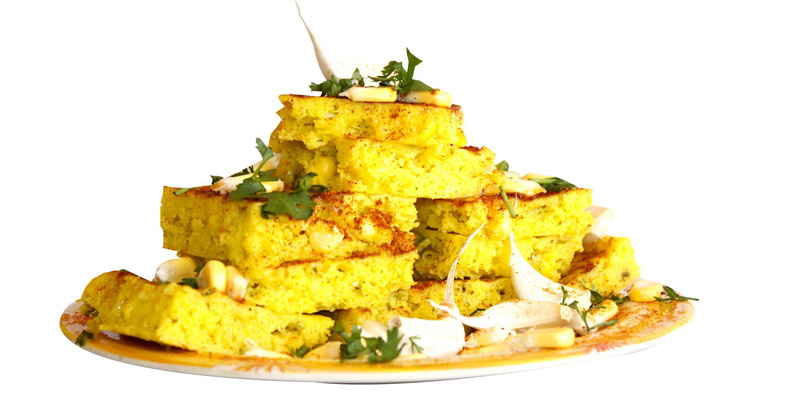# Mr. Dhokla

Street Food

### TIMING · Open Now

(Mon) 07:00 AM to 08:00 PM

D-3, Pramukh Darshan Appt., Chhatrapatri Shivaji Circle, Behind. Mahalaxmi Temple, Near. D Mart, Adajan, Surat

Find on Map

## Offers and Discount in Mr. Dhokla

Get 1 Plate Plain Dhokla (Rs. 25) + 1 Plate Oil Locho (Rs. 25) = Rs. 50 at Rs. 40

Get 2 Plates Dhokla (Rs. 25 X2 = Rs. 50) + 2 Plates of Masala Khaman (Rs. 20X2= Rs. 40) = (Rs. 90) at Rs. 80

Get 1 Plate Cheese Butter Dhokla (Rs. 50) + 1 Plate Cheese Butter Locho (Rs. 50) = (Rs. 100) at Rs. 80.

Get 2 Plates Live Dhokla (Rs. 20X2=Rs. 40) + 2 Plates Oil Locho (Rs. 25X2=Rs. 50) + 2 Plates Sev Khaman (Rs. 20X2= Rs. 40) = (Rs.130) at Rs.110.

Get 500gm Makai Dhokla (Rs. 70) + 500gm Vagharela Khaman (Rs. 90) + 500gm Vagharela Idada (Rs. 70 ) = (Rs.230) at Rs. 190/